# Texas Go Math Grade 8 Lesson 8.1 Answer Key The Pythagorean Theorem

Refer to our Texas Go Math Grade 8 Answer Key Pdf to score good marks in the exams. Test yourself by practicing the problems from Texas Go Math Grade 8 Lesson 8.1 Answer Key The Pythagorean Theorem.

## Texas Go Math Grade 8 Lesson 8.1 Answer Key The Pythagorean Theorem

Proving the Pythagorean Theorem

In a right triangle, the two sides that form the right angle are the legs. The side opposite the right angle is the hypotenuse.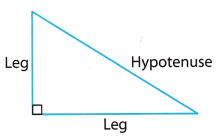The Pythagorean Theorem
In a right triangle, the sum of the squares of the lengths of the legs is equal to the square of the length of the hypotenuse.
If a and b are legs and c is the hypotenuse, a2 + b2 = c2.

A. Draw a right triangle on a piece of paper and cut it out. Make one leg shorter than the other.B. Trace your triangle onto another piece of paper four times, arranging them as shown. For each triangle, label the shorter leg a, the longer leg b, and the hypotenuse c.
C. What is the area of the unshaded square?
Label the unshaded square with its area.
D. Trace your original triangle onto a piece of paper four times again, arranging them as shown. Draw a line outlining a larger square that is the same size as the figure you made in B.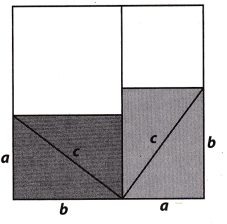E. What is the area of the unshaded square at the top right of the figure in D ? at the top left?
Label the unshaded squares with their areas.
F. What is the total area of the unshaded regions in D?

Reflect

Question 1.
Explain whether the figures in B and D have the same area.
We are told that the line outlining the larger square in figure D is the same as the one in figure B. In both of them, the side of the square is (a + b). Therefore, the figures in B and D have the same area.

Question 2.
Explain whether the unshaded regions of the figures in B and D have the same area.
We are told that the larger square in figure D is the same size as the one ¡n figure B. The shaded triangles in figure D are the same as the ones in figure B, but they are rearranged. Therefore, the unshaded regions of both figure D and B have the same area.

Question 3.
Analyze Relationships Write an equation relating the area of the unshaded region in step B to the unshaded region in D.
Taking into consideration figure B, the Legs of a single shaded triangle are denoted by a and b (where a < b) and the hypotenuse is denoted by c. As we can see, there are four equal shaded triangles and their hypotenuses form a square with side c. This means that the area of the unshaded region, denoted by A1, is:
A1 = c2
Taking into consideration figure D, we can notice again four shaded triangles with legs a and b and hypotenuse C, just like in figure B, but in this case the triangLes are rearranged. The area of the unshaded region can be found as the sum of the areas of the two unshaded square regions formed. The area of the first unshaded square, with side b, is given as b2, whether the area of the other unshaded square, with side a, is given as a2.
This means that the area of the unshaded region, denoted by A2, is:
A2 = a2 + b2
Since a and b are legs and c is the hypotenuse we know from the Pythagorean Theorem that a2 + b2 = c2.
Therefore,
A2 = a2+ b2 = c2
A2 = c2
As we can see A1 and A2 are equal.

Example 1

Find the length of the missing side.The length of the leg is 9 centimeters.

Find the length of the missing side.

Question 4.We use the Pythagorean Theorem to find the length of a side of a right triangle when we know the lengths of the other two sides.
a2 + b2 = c2 ………… (1)
302 + 402 = c2 (Substitute into the formula.) …… (2)
900 + 1600 = c2 (Simplify.) ………….. (3)
2500 = c2 (Add) …………… (4)
50 = c (Take the square root of both sides.) ……………. (5)

Question 5.We use the Pythagorean Theorem to find the length of a side of a right triangle when we know the lengths of the other two sides.
a2 + b2 = c2 …………… (1)
a2 + 402 = 412 (Substitute into the formula.) ………….. (2)
a2 + 1600 = 1681 (Simplify.) …………… (3)
a2 = 81 (Use properties of equality to get a2 by itself.) ……………. (4)
a = 9 (Take the square root of both sides.) ……………… (5)

Question 6.
Tina ordered a replacement part for her desk. It was shipped in a box that measures 4 in. by 4 in. by 14 in. What is the greatest length, in whole inches, that the part could have been?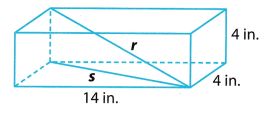We want to find r, the length from a bottom corner to the opposite top corner. First, we find s, the length of the diagonal across the bottom of the box.
w2 + l2 = s2
42 + 142 = s2
16 + 196 = s2
212 = s2
We use our expression for s to find r.
h2 + s2 = r2
42 + 212 = r2
16 + 212 = r2
228 = r2
15.1 ≈ r
The greatest length in whole inches that the part could have been is 15 inches.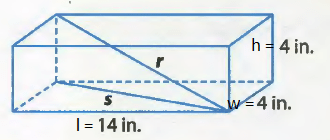Question 1.
Find the length of the missing side of the triangle. (Explore Activity 1 and Example 1)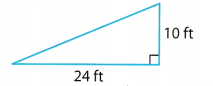a2 + b2 = c2 → 242 + ______ = c2 → ________ = c2
The length of the hypotenuse is ________ feet.
a2 + b2 = c2
242 + 102 = c2
576 + 100 = c2
676 = c2
c = 26
The length of the hypotenuse is 26 feet

Question 2.
Mr. Woo wants to ship a fishing rod that is 42 inches long to his son. He has a box with the dimensions shown. (Example 2)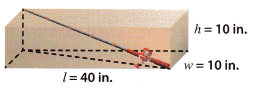a. Find the square of the length of the diagonal across the bottom of the box.
We denote by s, the length of the diagonal across the bottom of the box.
w2 + l2 = s2
402 + 102 = s2
1600 + 100 = s2
1700 = s2

b. Find the length from a bottom corner to the opposite top corner to the nearest tenth. Will the fishing rod fit?
We denote by r, the length from the bottom corner to the opposite top corner. We use our expression for s to find r.
h2 + s2 = r2
102 + 1700 = r2
100 + 1700 = r2
1800 = r2
42.2 ≈ r
Since the fishing rod is 42 inches (smaller than r), it will fit in the box.

Essential Question Check-In

Question 3.
Use a model or a diagram to help you state the Pythagorean Theorem and tell how you can use it to solve problems.
Pythagorean Theorem
In a right triangle, the sum of squares of the legs a and b is equal to the square of the hypotenuse c.
a2 + b2 = c2
You can use it to find the length of a side of a right triangle when the lengths of the other two sides are known.

Find the length of the missing side of each triangle. Round your answers to the nearest tenth.

Question 4.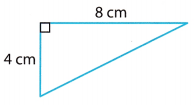Using the Pythagorean Theorem we find the length of a side of a right triangle when we know the lengths of the other two sides.
a2 + b2 = c2 ……………. (1)
42 + 82 = c2 (Substitute into the formula.) ………… (2)
16 + 64 = c2 (Simplify.) …………. (3)
80 = c2 (Add) …………… (4)
(Take the square root of both sides.) ………… (5)
c = 8.9442 (Calculate) ………… (6)
c ≈ 8.9 (Round to the nearest tenth) …………. (7)
c = 8.9 cm

Question 5.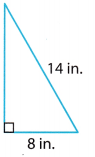Using the Pythagorean Theorem we have:
a2 + b2 = c2
a2 + 82 = 142
a2 + 64 = 196
a2 + 64 – 64 = 196 – 64
a2 = 132
We are told to round our answer to the nearest tenth, therefore:
a ≈ 11.5Question 6.
The diagonal of a rectangular TV screen measures 152 cm. The length measures 132 cm. What is the height of the screen?
The diagonal of a rectangular TV screen is d = 15 2cm. The length is l = 132 cm. Let’s find the measure of the height h.
As t and h are two sides that form the right angle of a right triangle with a hypotenuse d, we can use the
Pythagorean Theorem to find the length of height.
d2 = l2 + h2 ………………. (1)
1522 = 1322 + h2 (Substitute) …………. (2)
h2 = 23104 – 17424 (Use properties of equaLity to get h2 by itself.) ………… (3)
h2 = 5680 (Calculate) …………… (4)
h = 75.3658 (Take the square root of both sides.) ……………. (5)
h ≈ 75.4 (Round to the nearest tenth) ……………. (6)
Height is approx 75.4 cm

Question 7.
Dylan has a square piece of metal that measures 10 inches on each side. He cuts the metal along the diagonal, forming two right triangles. What is the length of the hypotenuse of each right triangle to the nearest tenth of an inch?
Using the Pythagorean Theorem, we have:
a2 + b2 = c2
102 + 102 = c2
100 + 100 = c2
200 = c2
We are told to round the length of the hypotenuse of each right triangle to the nearest tenth of an inch, therefore:
c ≈ 14.11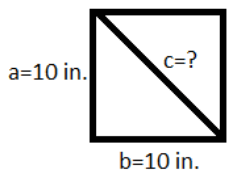Question 8.
Represent Real-World Problems A painter has a 24-foot ladder that he is using to paint a house. For safety reasons, the ladder must be placed at least 8 feet from the base of the side of the house. To the nearest tenth of a foot, how high can the ladder safely reach?
If we denote with a the height that the ladder can reach, with b the length of the ladder from the base of the side of the house and with c the length of the ladder, using the Pythagorean Theorem, we have:
a2 + b2 = c2
a2 + 82 = 242
a2 + 64 = 756
a2 = 512
We are toLd to round the length to the nearest tenth of a foot, therefore:
a ≈ 22.6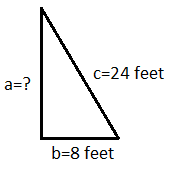Question 9.
What is the longest flagpole (in whole feet) that could be shipped in a box that measures 2 ft by 2 ft by 12 ft? ___________We want to find r, the length from a bottom corner to the opposite top corner. First, we find s, the length of the diagonal across the bottom of the box.
w2 + l2 = s2
22 + 122 = s2
4 + 144 = s2
148 = s2
We use our expression for s to find r.
h2 + s2 = r2
22 + 148 = r2
4 + 148 = r2
152 = r2
12.33 ≈ r
The longest flagpole (in whole feet) that could be shipped in this box is 12 inches.Question 10.
Sports American football fields measure 100 yards long between the end zones, and are 53 yards wide, Is the length of the diagonal across this field more or less than 120 yards? Explain.
We are given l = 100 and w = 53$$\frac{1}{3}$$ = $$\frac{160}{3}$$. If we denote with d the diagonal of the field, using the Pythagorean Theorem, we have:
l2 + w2 = d2
1002 + ($$\frac{160}{3}$$)2 = d2
10000 + $$\frac{25600}{9}$$ = d2
9 ∙ 10000 + 9 ∙ $$\frac{25600}{9}$$ = 9 ∙ d2
90000 + 25600 = 9 ∙ d2
115600 = 9 ∙ d2
$$\frac{115600}{9}$$ = d2
$$\frac{340}{3}$$ = d
d = $$113 . \overline{3}$$
The diagonal across this field is less than 120 yards.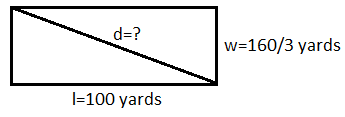Question 11.
Justify Reasoning A tree struck by lightning broke at a point 12 ft above the ground as shown. What was the height of the tree to the nearest tenth of a foot? Explain your reasoning.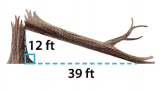Using the Pythagorean Theorem, we have:
a2 + b2 = c2
122 + 392 = c2
144 + 1521 = c2
1665 = c2
We are told to round the length of the hypotenuse to the nearest tenth of a foot, therefore:
c ≈ 40.8
Therefore, the total height of the tree was:
height = a + c
height = 12 + 40.8
height = 52.8The total height of the tree was 52.8 feet.

H.O.T. Focus on Higher Order Thinking

Question 12.
Multistep Main Street and Washington Avenue meet at a right angle. A large park begins at this corner. Usually, Joe walks 1.2 miles along Main Street and then 0.9 miles up Washington Avenue to get to school. Today he walked in a straight path across the park and returned home along the same path. What is the difference in distance between Joe’s round trip today and his usual round trip? Explain.
Using the Pythagorean Theorem, we find the distance from his home to school following the straight path across
the park:
a2 + b2 = c2
1.22 + 0.92 = c2
1.44 + 0.81 = c2
2.25 = c2
1.5 = c
Therefore, the distance of Joe’s round trip following the path across the park is 3 mites (dhome – school + dschool – home = 1.5 + 1.5). Usually, when he walks aLong Main Street and Washington Avenue, the distance of his round trip is 4.2 miles (dhome – school + dschool – home = (1.2 + 0.9) + (0.9 + 1.2)). As we can see, Joe walks 1.2 miles less if he follows the straight path across the park.

Question 13.
Analyze Relationships An isosceles right triangle is a right triangle with congruent legs. If the length of each leg is represented by x, what algebraic expression can be used to represent the length of the hypotenuse? Explain your reasoning.
From the Pythagorean Theorem, we know that if a and b are legs and c is the hypotenuse, then a2 + b2 = c2. In our case, the length of each leg is represented by x, therefore we have:
a2 + b2 = c2
x2 + x2 = cc
2x2 = c2
c = x$$\sqrt {2}$$

Question 14.
Persevere in Problem Solving A square hamburger is centered on a circular bun. Both the bun and the burger have an area of 16 square inches.a. How far, to the nearest hundredth of an inch, does each corner of the burger stick out from the bun? Explain.
First, we need to find the radius r of the circular bun. We know that its area A is 16 square inches, therefore:
A = πr2
16 = 3.14 ∙ r2
r2 = $$\frac{16}{3.14}$$
r ≈ 3.14
Then, we need to find the side s of the square hamburger. We know that its area A is 16 square inches, therefore:
A = s2
16 = s2
s = 4
Using the Pythagorean Theorem, we have to find the diagonal d of the square hamburger:
s2 + s2 = d2
42 + 42 = d2
16 + 16 = d2
32 = d2
d ≈ 5.66
To find how far does each corner of the burger stick out from the bun, we denote this length by a and we get:
a = $$\frac{d}{2}$$ – r = $$\frac{5.66}{2}$$ – 2.26
a = 0.57
Each corner of the burger stick out 0.57 inches from the bun.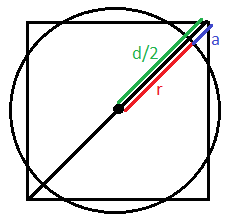b. How far does the bun stick out from the center of each side of the burger?
b = r – $$\frac{s}{2}$$ = 2.26 – $$\frac{4}{2}$$